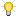Operators
Most of the operators used in trigger scripts are similar to other programming languages; however, there are some slight differences. For example, there is a fundamental difference between the assignment operator “=“ and the comparison operator “==“. Be careful not to use “=“ to mean “equal to” in a comparison statement.The string operator “+” is very useful for constructing error and log messages in trigger scripts. The following tables outlines operators used in script triggers:
 Arithmetic Operators Comparison Operators Addition: + Subtraction: - Multiplication: * Division: / Modulus: % Increment: ++ Decrement: -- Is equal to: == Is not equal to: != Greater than: > Greater than or equal to: >= Less than: < Less than or equal to: <=
 Logical Operators String Operators And: && Or: || Not: ! Assignment: = Concatenate: + Concatenate and assign: +=